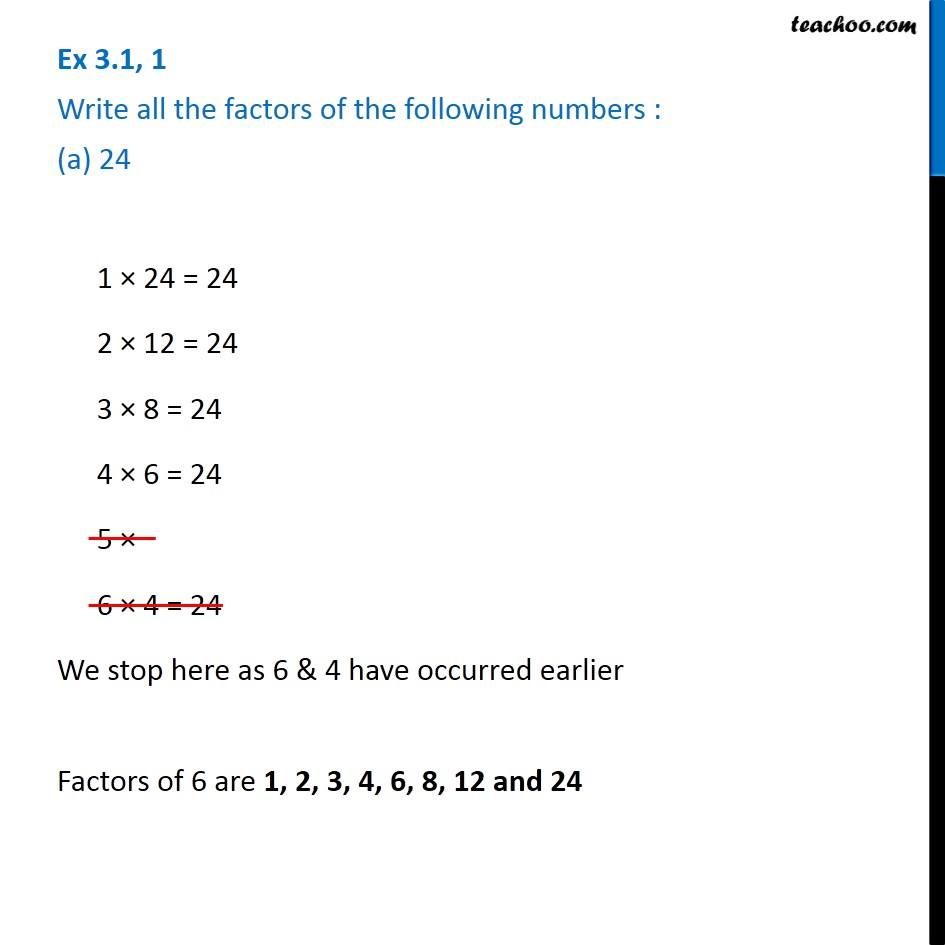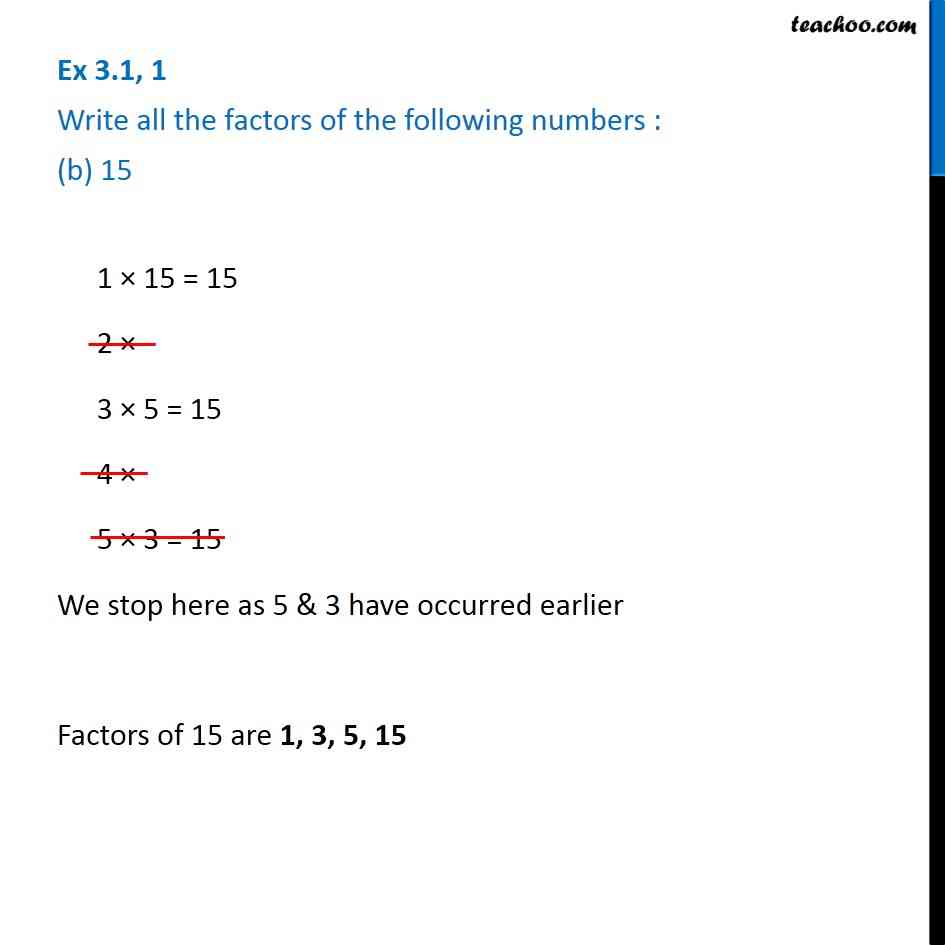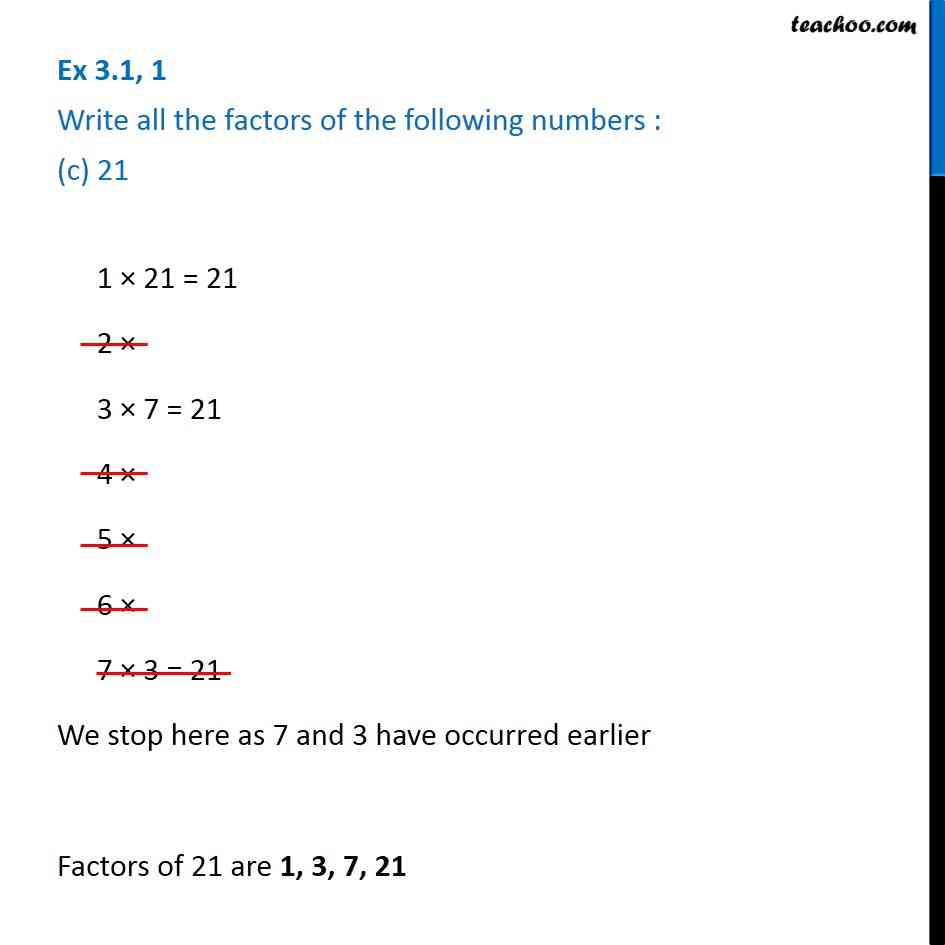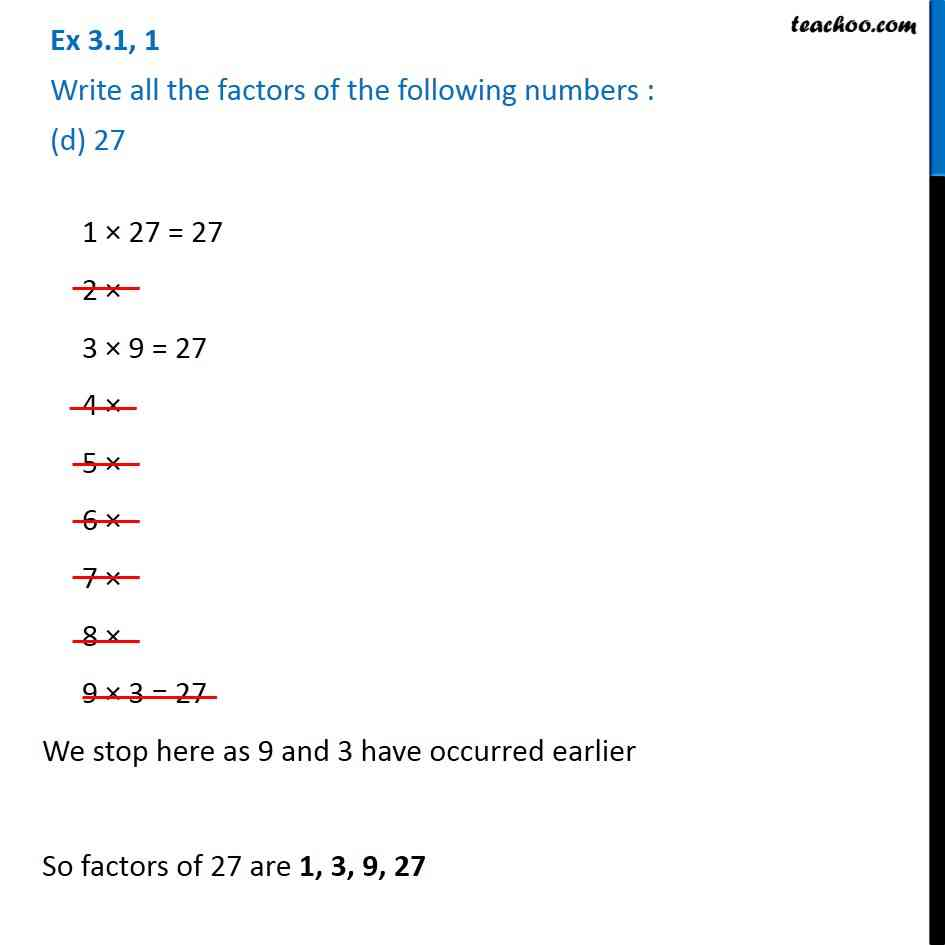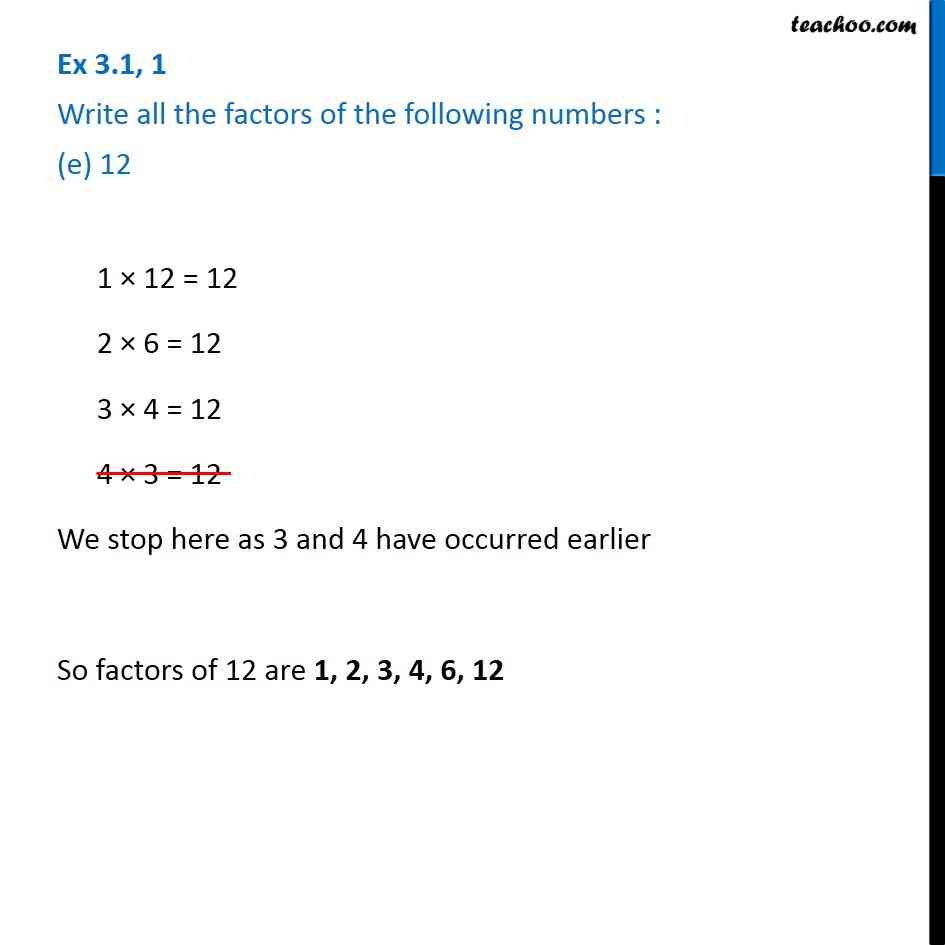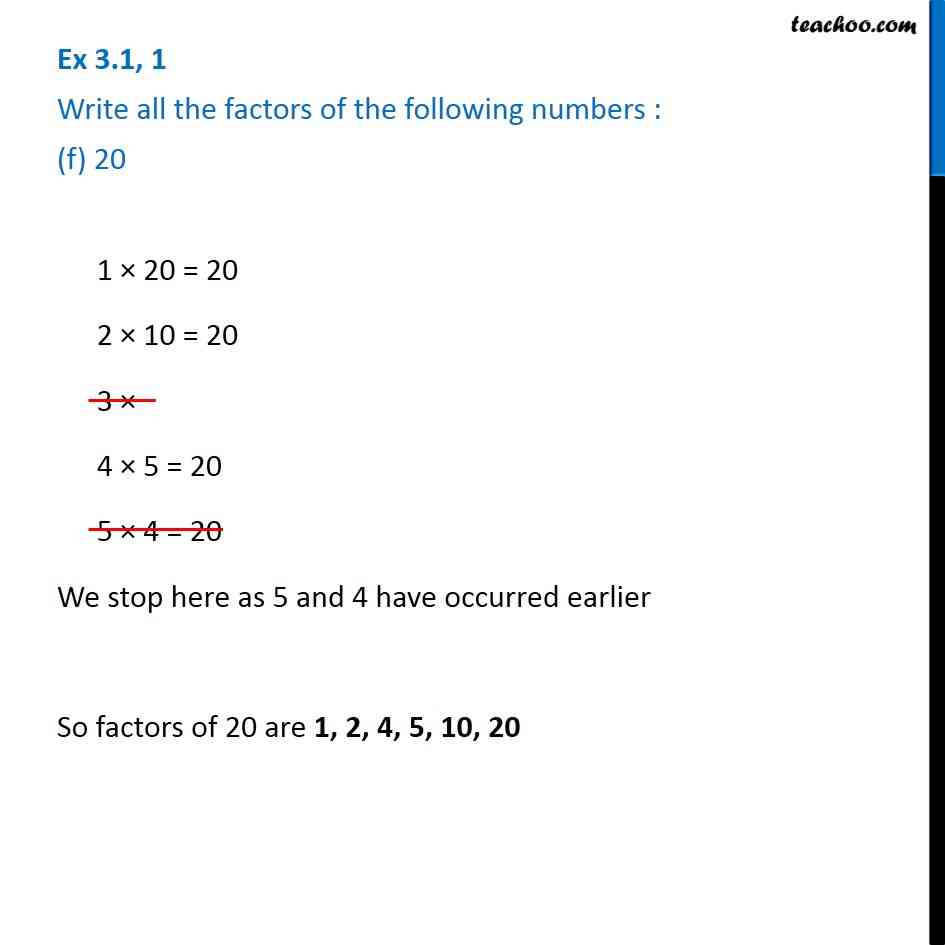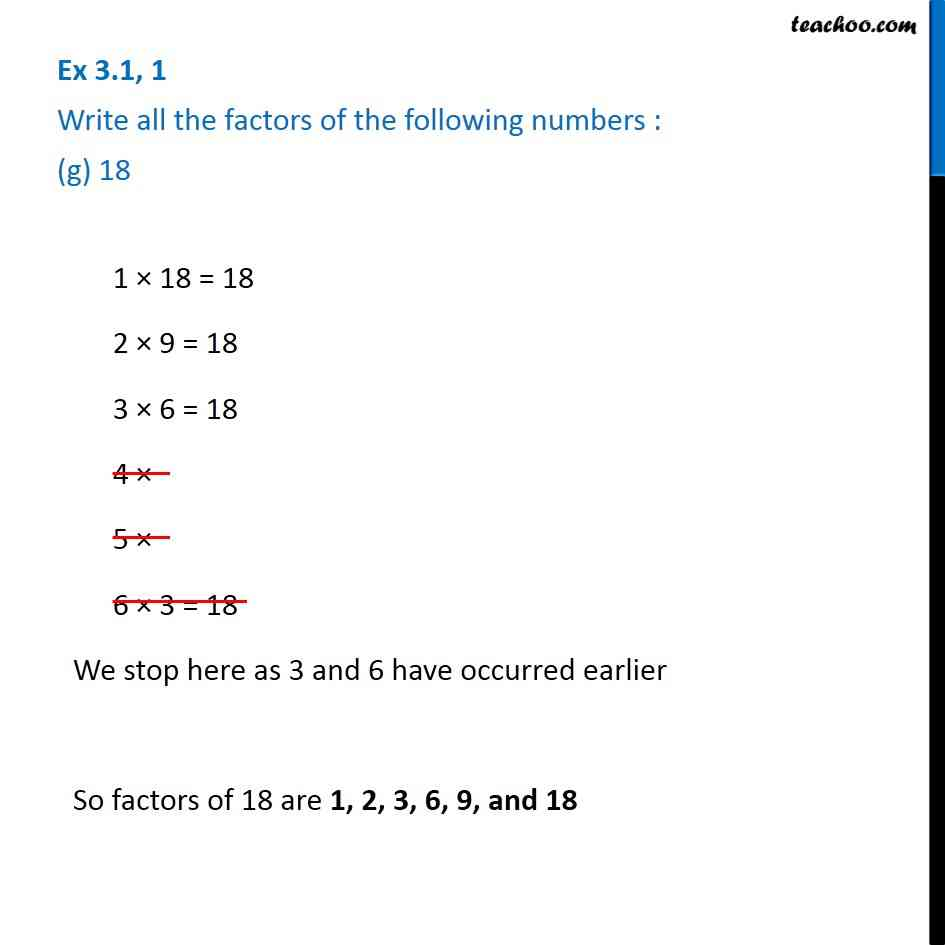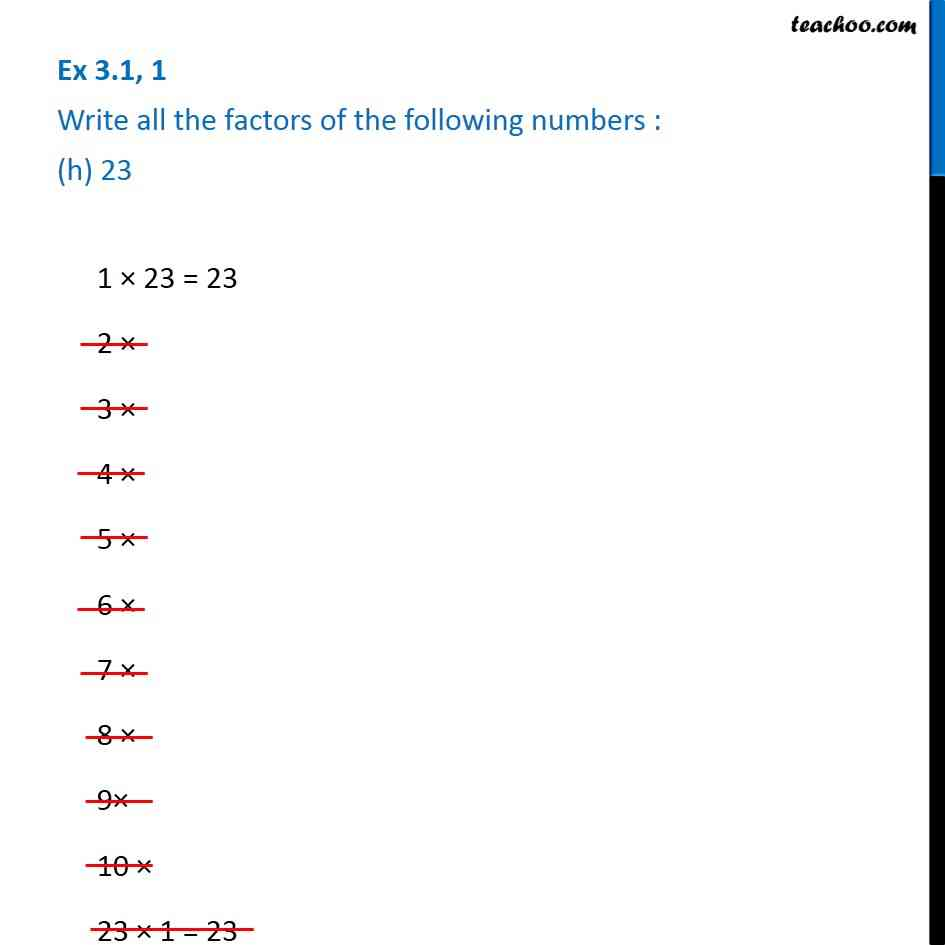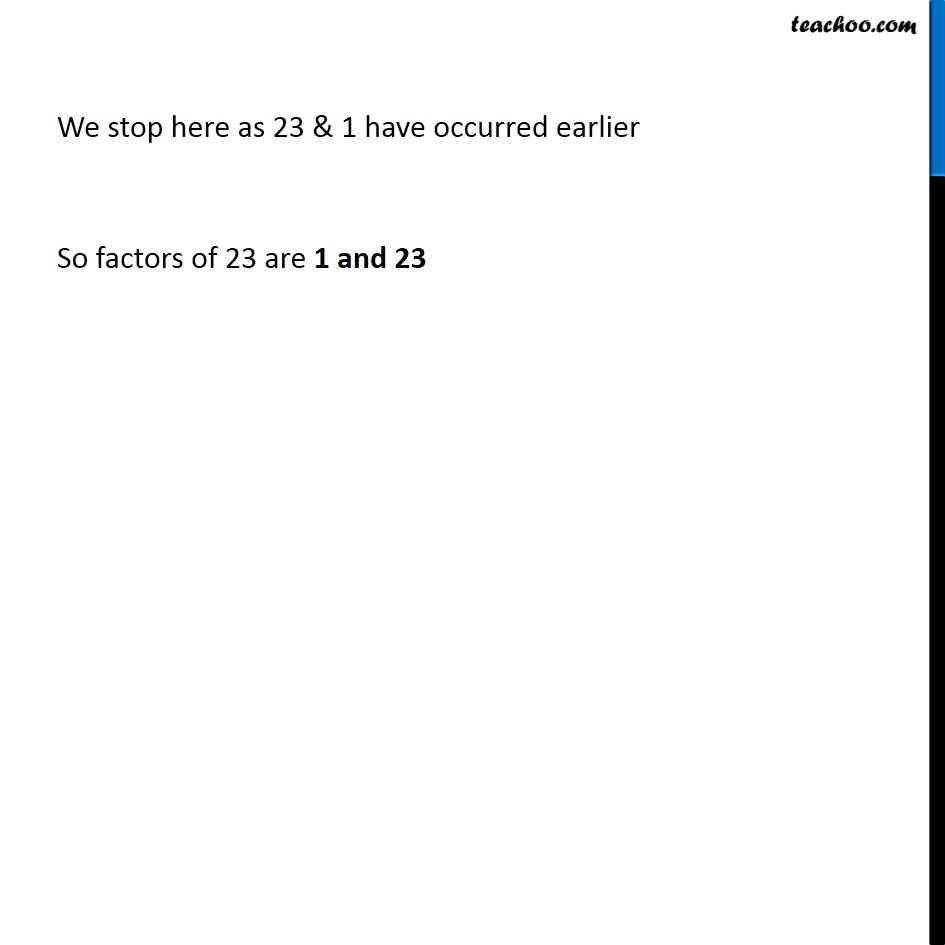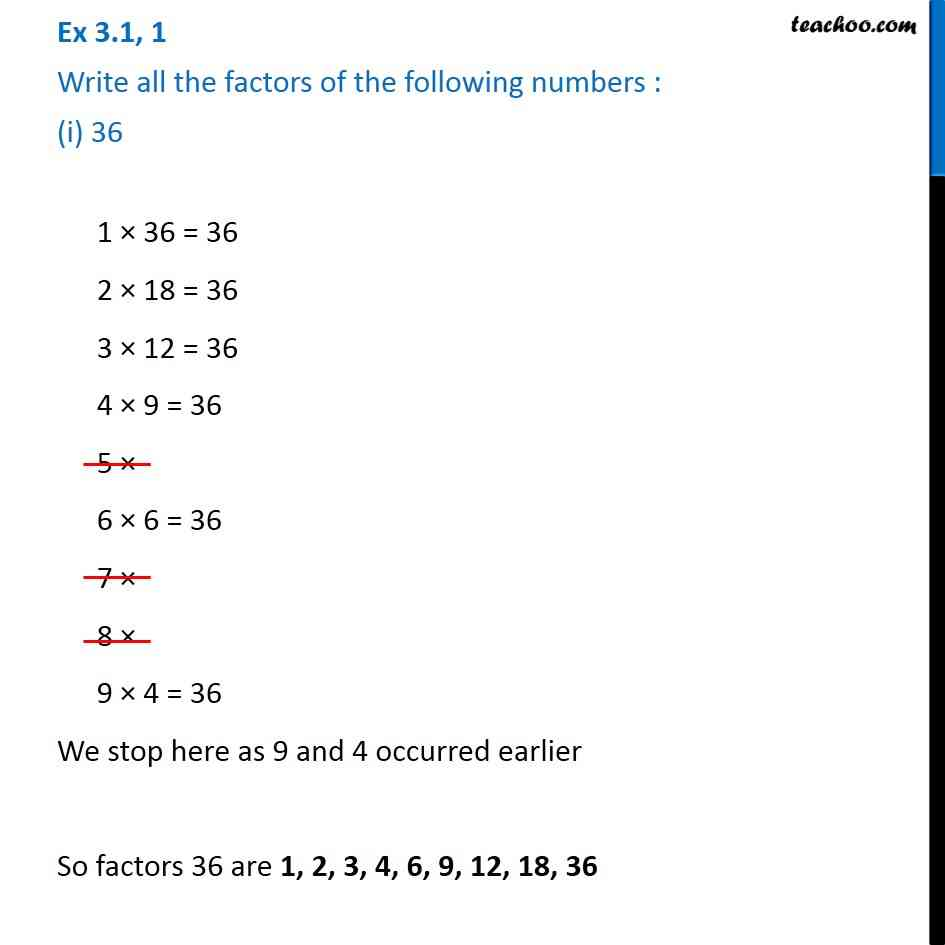Subscribe to our Youtube Channel - https://you.tube/teachoo

1. Chapter 3 Class 6 Playing with Numbers
2. Serial order wise
3. Ex 3.1

Transcript

Ex 3.1, 1 Write all the factors of the following numbers : (a) 24 1 × 24 = 24 2 × 12 = 24 3 × 8 = 24 4 × 6 = 24 5 × 6 × 4 = 24 We stop here as 6 & 4 have occurred earlier Factors of 6 are 1, 2, 3, 4, 6, 8, 12 and 24 Ex 3.1, 1 Write all the factors of the following numbers : (b) 15 1 × 15 = 15 2 × 3 × 5 = 15 4 × 5 × 3 = 15 We stop here as 5 & 3 have occurred earlier Factors of 15 are 1, 3, 5, 15 Ex 3.1, 1 Write all the factors of the following numbers : (c) 21 1 × 21 = 21 2 × 3 × 7 = 21 4 × 5 × 6 × 7 × 3 = 21 We stop here as 7 and 3 have occurred earlier Factors of 21 are 1, 3, 7, 21 Ex 3.1, 1 Write all the factors of the following numbers : (d) 27 1 × 27 = 27 2 × 3 × 9 = 27 4 × 5 × 6 × 7 × 8 × 9 × 3 = 27 We stop here as 9 and 3 have occurred earlier So factors of 27 are 1, 3, 9, 27 1 × 27 = 27 2 × 3 × 9 = 27 4 × 5 × 6 × 7 × 8 × 9 × 3 = 27 We stop here as 9 and 3 have occurred earlier So factors of 27 are 1, 3, 9, 27 Ex 3.1, 1 Write all the factors of the following numbers : (e) 12 1 × 12 = 12 2 × 6 = 12 3 × 4 = 12 4 × 3 = 12 We stop here as 3 and 4 have occurred earlier So factors of 12 are 1, 2, 3, 4, 6, 12 Ex 3.1, 1 Write all the factors of the following numbers : (f) 20 1 × 20 = 20 2 × 10 = 20 3 × 4 × 5 = 20 5 × 4 = 20 We stop here as 5 and 4 have occurred earlier So factors of 20 are 1, 2, 4, 5, 10, 20 Ex 3.1, 1 Write all the factors of the following numbers : (g) 18 1 × 18 = 18 2 × 9 = 18 3 × 6 = 18 4 × 5 × 6 × 3 = 18 We stop here as 3 and 6 have occurred earlier So factors of 18 are 1, 2, 3, 6, 9, and 18 1 × 18 = 18 2 × 9 = 18 3 × 6 = 18 4 × 5 × 6 × 3 = 18 We stop here as 3 and 6 have occurred earlier So factors of 18 are 1, 2, 3, 6, 9, and 18 Ex 3.1, 1 Write all the factors of the following numbers : (h) 23 1 × 23 = 23 2 × 3 × 4 × 5 × 6 × 7 × 8 × 9× 10 × 23 × 1 = 23 We stop here as 23 & 1 have occurred earlier So factors of 23 are 1 and 23 Ex 3.1, 1 Write all the factors of the following numbers : (i) 36 1 × 36 = 36 2 × 18 = 36 3 × 12 = 36 4 × 9 = 36 5 × 6 × 6 = 36 7 × 8 × 9 × 4 = 36 We stop here as 9 and 4 occurred earlier So factors 36 are 1, 2, 3, 4, 6, 9, 12, 18, 36

Ex 3.1